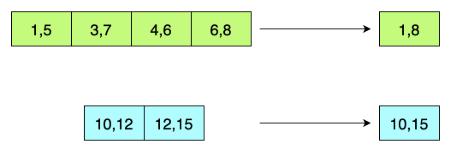# Merge Overlapping Intervals

## Problem Statement

You are given an array (list) of interval pairs as input where each interval has a start and end timestamp. The input array is sorted by starting timestamps. You are required to merge overlapping intervals and return a new output array.

Consider the input array below. Intervals (1, 5), (3, 7), (4, 6), (6, 8) are overlapping so they should be merged to one big interval (1, 8). Similarly, intervals (10, 12) and (12, 15) are also overlapping and should be merged to (10, 15).## Hint

• Try the linear scan
• Use the pair class defined in the code below to handle pairs of time stamps

## Try it yourself

class Pair{  public:    int first, second;    Pair(int x, int y){      first = x;      second = y;     }};vector<Pair> merge_intervals(vector<Pair>& v){  vector<Pair> result;  // write your code here  return result; }

## Solution

class Pair{  public:    int first, second;    Pair(int x, int y){      first = x;      second = y;     }};vector<Pair> merge_intervals(vector<Pair>& v) {  if(v.size() == 0) {    return v;  }  vector<Pair> result;  result.push_back(Pair(v.first, v.second));  for(int i = 1 ; i < v.size(); i++){    int x1 = v[i].first;    int y1 = v[i].second;    int x2 = result[result.size() - 1].first;    int y2 = result[result.size() - 1].second;    if(y2 >= x1){      result[result.size() - 1].second = max(y1, y2);    }    else{      result.push_back(Pair(x1, y1));    }  }  return result;}int main() {  vector<Pair> v {                  Pair(1, 5),                  Pair(3, 7),                  Pair(4, 6),                  Pair(6, 8),                  Pair(10, 12),                  Pair(11, 15)                  };  vector<Pair> result = merge_intervals(v);    for(int i = 0; i < result.size(); i++){    cout << "[" << result[i].first << ", " << result[i].second << "] ";  }}

## Solution Explanation

### Runtime complexity

The runtime complexity of this solution is linear, O(n).

### Memory complexity

The memory complexity of this solution is linear, O(n).

### Solution Breakdown

This problem can be solved in a simple linear scan algorithm. We know that input is sorted by starting timestamps. Here is the approach we are following:

• List of input intervals is given, and we’ll keep merged intervals in the output list.

• For each interval in the input list:

• If the input interval is overlapping with the last interval in output list then we’ll merge these two intervals and update the last interval of output list with merged interval.
• Otherwise, we’ll add an input interval to the output list.

Practice problems like this and many more by checking out our Grokking the Coding Interview: Patterns for Coding Questions course!

Learn in-demand tech skills in half the time